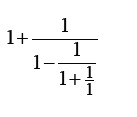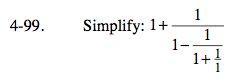### Home > PC > Chapter 4 > Lesson 4.2.3 > Problem4-99

4-99.

Simplyfy:Homework Help ✎Start with the lowest fraction getting common denominators and simplifying as you work up.

$=1+\frac{1}{1-\frac{1}{2}}=1+\frac{1}{\frac{1}{2}}=1+2=3$